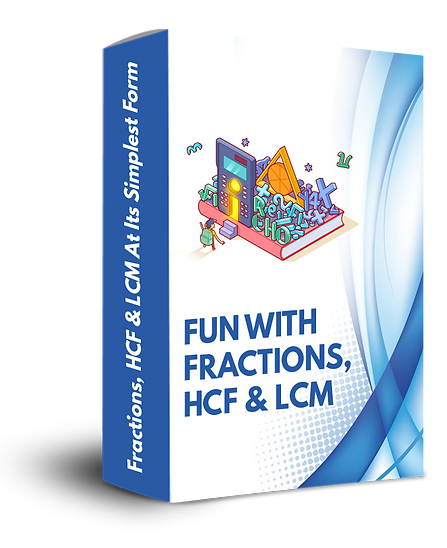top of page## There is a fine line between Numerator & Denominator. Understanding Matters!!

### Quiz after every chapter

Description:

Here we have 3 Topics explained in detail, lets's see what are they:-

We have Fractions, HCF and LCM

A- Fractions:-

Fractions are nothing but having a number on top and a number on the bottom with a line between the two numbers. Here the number on top is the Numerator and the number on the bottom is called the Denominator. We use the Vertically and Crosswise formula.

Under Fractions we have 2 Cases:-
1- When the denominators are Relatively Prime
2- When the denominators are Composite Numbers.

B- HCF / LCM

Here we have easy tricks to solve Average, HCF and LCM.
Most commonly we use the division method or prime factorization method to find the Highest Common Factor(HCF).
The Lowest Common Multiple(LCM) of 2 or more numbers is the smallest number(not zero) that is a multiple of all the given numbers.
All the above topics give easy ways to calculate and find answers in very simple methods.
The procedures of finding answers are made shorter and few tips have been given.## Mrs. Nandini, Teacher

The Beginners Guide is the best course for students to learn basics of Vedic Maths and it encourages to calculate mentallyTricks are very good and it makes my kid think the logic behind every answer. Looking forward to the other courses in Roadmap## Mrs. Rekha, Proud Mother

After every chapter my son takes the exercise problems as a challenge and works in the same pattern as thought. A great course!bottom of page